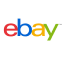New update is available. Click here to update.
Last Updated: 21 May, 2021

# Party Over

Easy## Problem statement#### Ninja gets totally confused, he asks you to solve the problem. Can you help Ninja figure out the correct order of strings?

##### Input Format:
``````The first line of input contains an integer ‘T,’ denoting the number of test cases. The test cases follow.

The first line of each test case contains a number ‘n’ denoting the number of strings.

The second line of each test case contains ‘n’ space-separated strings that the monster gave to Ninja.
``````
##### Output Format:
``````For each test case, print the strings sorted according to the last character.

Print the output of each test case in a separate line.
``````
##### Note:
``````You are not required to print the expected output; it has already been taken care of. Just implement the function.
``````
##### Constraints:
``````1 <= T <= 10
1 <= n <= 10^3
1 <= size of string <= 10^2

Where 'T’ is the number of test cases, ‘n’ denotes the number of strings

Time Limit: 1 sec
``````## Approaches

### 01 ApproachThe idea is to reverse all the strings and then sort them in alphabetical order and return the sorted strings back in their original form. We can use a nested loop and compare each string with all the preceding strings to sort the strings.

The steps are as follows:

• Initially, input a string array “arr” containing all the strings needed to be sorted based on the last character.
• Reverse the array containing all the strings using the in-built reverse() function.
• We will apply a nested loop with the outer loop pointed by ‘i’ starting from 0 and going till ‘n’ - 1 where ‘n’ is the number of strings in the array and the inner loop pointed by j=i+1 and going till ‘n.’
• Now we will start comparing the strings arr[i] and arr[j] using the relational operators.
• We will check if the compare() function returns a value greater than 0. If it does, then we can be sure that arr[i] is lexicographically more significant than the string arr[j].
• Hence swap both strings using the in-built swap function.
• After the swapping operation is performed, reverse back all the strings in their original form.
• We will return the array containing all the strings as the answer.